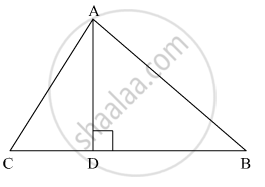# The perpendicular from A on side BC of a Δ ABC meets BC at D such that DB = 3CD. Prove that 2AB2 = 2AC2 + BC2. - Mathematics

Sum

The perpendicular from A on side BC of a Δ ABC meets BC at D such that DB = 3CD. Prove that 2AB2 = 2AC+ BC2.

#### SolutionApplying Pythagoras theorem for ΔACD, we obtain

AD = AC2 - DC2 ......(1)

Applying Pythagoras theorem in ΔABD, we obtain

AD2 = AB2 - DB2 .....(2)

From equation (1) and equation (2), we obtain

AC2 - DC2 = AB2 - DB2 .....(3)

It is given that 3DC = DB

∴ "DC" = "BC"/4 and "DB"=(3"BC")/ 4

Putting these values in equation (3), we obtain

"AC"^2 - ("BC"/4)^2 = "AB"^2 - ((3"BC")/4)^2

"AC"^2 - "BC"^2/16 = "AB"^2 - (9"BC"^2)/4

16AC2 - BC2 = 16AB2 - 9BC2

16AB2 - 16AC2 = 8BC2

2AB2 = 2AC2 + BC2

Concept: Areas of Similar Triangles
Is there an error in this question or solution?